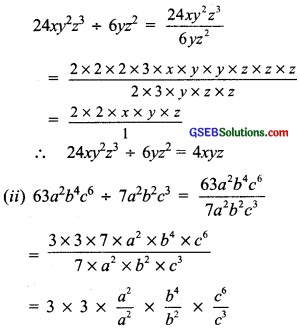# GSEB Solutions Class 8 Maths Chapter 14 Factorization Intex Questions

Gujarat Board GSEB Textbook Solutions Class 8 Maths Chapter 14 Factorization Intex Questions and Answers.

## Gujarat Board Textbook Solutions Class 8 Maths Chapter 14 Factorization Intex Questions

Try These (Page 219)

Question 1.
Factorise

1. 12x + 36
2. 22y – 33z
3. 14pq + 35pqr

Solution:
1. We have 12x = 3 × 2 × 2 × x
= (2 × 2 × 3) × x
36 = 2 × 2 × 3 × 3
= (2 × 2 × 3) × 3
∴ 12x + 36 = [(2 × 2 × 3) × x] + (2 × 2 × 3) × 3
= (2 × 2 × 3)[x + 3]
= 12[x + 3]

2. We have 22y = 2 × 11 × y
33z = 3 × 11 × z
∴ 22y – 33z = [2 × (11) × y] – [3 × (11) × z]
= (11)[2 × y – 3 × z]
= 11[2y – 3z]

3. We have 14pq = 2 × 7 × p × q = 2 × (7 × p × q)
35pqr = 5 × 7 × p × q × r
= 5 × (7 × p × q) × r
∴ 14pq + 35pqr
= 2 × (7 × p × q) + 5 × (7 × p × q) × r
= (7 × p × q)[2 + 5 × r] = 7pq(2 + 5r)Try These (Page 225)

Question 1.
Divide:
(i) 24xy2z3 by 6yz2
(ii) 63a2b4c6 by 7a2b2c3
Solution:
(i) We have 2xy2z3= 9 × a2-2 × b4-2 × c6-3
= 9 × a0 × b2 × c3
= 9 × 1 × b2 × c3 = 9b2c3
∴ 63a2b4c6 ÷ 7a2b2c3 = 9b2c3